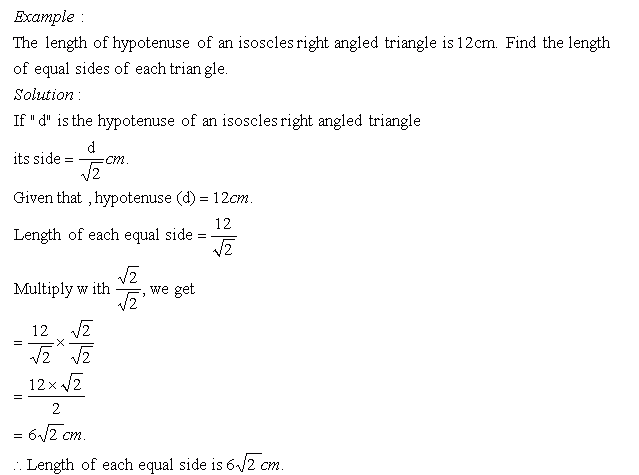Name: ___________________Date:___________________

 Email us to get an instant 20% discount on highly effective K-12 Math & English kwizNET Programs!

### Grade 8 - Mathematics3.19 Isosceles Right Triangle - 2Directions: Solve the following problems. Also write at least ten examples of your own.
 Q 1: The length of hypotenuse of an isosceles right angled triangle is 9Ö2 cm. Find the length of equal sides of triangle.9None of these9Ö2 cm Q 2: The length of hypotenuse of an isosceles right angled triangle is 16Ö2 cm. Find the length of equal sides of triangle.16Ö2 cm16None of these Q 3: The length of hypotenuse of an isosceles right angled triangle is 8 cm. Find the length of equal sides of triangle.4.5Ö2 cm5Ö2 cm5.5Ö2 cm4Ö2 cm. Q 4: The length of hypotenuse of an isosceles right angled triangle is 8Ö2 cm. Find the length of equal sides of triangle.88Ö2 cmNone of these Q 5: The length of hypotenuse of an isosceles right angled triangle is 72 cm. Find the length of equal sides of triangle.32Ö2 cm34Ö2 cm36Ö2 cm35Ö2 cm Q 6: The length of hypotenuse of an isosceles right angled triangle is 50 cm. Find the length of equal sides of triangle.None of these23.5Ö2 cm25Ö2 cm20Ö2 cm Q 7: The length of hypotenuse of an isosceles right angled triangle is 15Ö2 cm. Find the length of equal sides of triangle.15None of these15Ö2 cm Q 8: The length of hypotenuse of an isosceles right angled triangle is 18 cm. Find the length of equal sides of triangle.11Ö2 cm9Ö2 cm10Ö2 cm8Ö2 cm Question 9: This question is available to subscribers only! Question 10: This question is available to subscribers only!

#### Subscription to kwizNET Learning System costs less than \$1 per month & offers the following benefits:

• Unrestricted access to grade appropriate lessons, quizzes, & printable worksheets
• Instant scoring of online quizzes
• Progress tracking and award certificates to keep your student motivated
• Unlimited practice with auto-generated 'WIZ MATH' quizzes